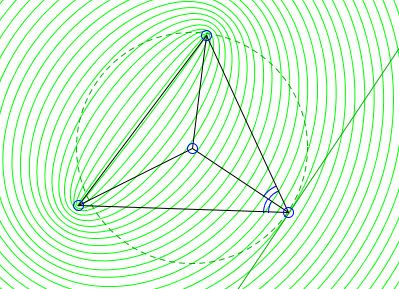# Maximum Perimeter Property of the Incircle

Given point $P,\,$ points $A,\,$ $B,\,$ $C\,$ are at fixed distances $AP,\,$ $BP,\,$ and $CP\,$ from $P.\,$ Among all triangles $ABC,\,$ the one for which $P\,$ is the incenter, has the maximum perimeter.

The claim amounts to the assertion that, for the optimal $\Delta ABC,\,$ $P\,$ lies on the bisectors of all three angles of the triangle. The applet below illustrates the fact that no other triangle may possibly has the maximum perimeter.

In the applet, point $P\,$ always moves freely. Points $A,\,$ $B,\,$ $C\,$ move on circles centered at $P.\,$

When one of the points $A,\,$ $B,\,$ or $C\,$ is being dragged, the applet displays the circle to which the point is constrained. Assume $A\,$ is being dragged. In this case, $BC\,$ is constant so that the change in the magnitude of the perimeter $AB + BC + CA\,$ is reflected in the change of the sum $f(A) = AB + CA.\,$ This sum is constant on the ellipses with the foci at $B\,$ and $C.\,$ Let $A\,$ move on a circle $C(P),\,$ with center $P.\,$ Function $f(A)\,$ cannot have a (local) maximum wherever $C(P)\,$ intersects the ellipses, but only when it is tangent to one of them. In principle, there are two such ellipses. At the point of tangency with one of them $f(A)\,$ attains a global maximum.At the points of tangency, $C(P)\,$ shares the tangent with the corresponding ellipse. But ellipse is known to possess the mirror property: the lines joining the foci to a point on the ellipse form equal angles with the tangent, meaning, in particular, that the perpendicular to the tangent at that point serves as the bisector of angle $BAC.\,$ But the perpendicular to a tangent on a point of a circle is a radius of the circle, so that, for such point $A\,$ where $C(P)\,$ is tangent to on of the ellipses, $AP\,$ is the bisector of angle $BAC.\,$ When this happens, the applet displays the ellipse and the common tangent.

Created with GeoGebra

The function $f(A, B, C) = AB + BC + CA\,$ is a continuous function of its arguments. The Cartesian product of three circles with radii $AP,\,$ $BP,\,$ $CP\,$ centered at $P\,$ is a compact set so that the function does attain its maximum. For the corresponding triangle $ABC,\,$ $AP,\,$ $BP,\,$ and $CP\,$ must be angle bisectors of angles $BAC,\,$ $ABC,\,$ $ACB,\,$ respectively, implying that $P\,$ is the incenter of that triangle.## Ellipse### A Sample of Optimization Problems II

• Mathematicians Like to Optimize
• Building a Bridge
• Building Bridges
• Optimization Problem in Acute Angle
•